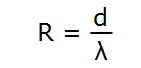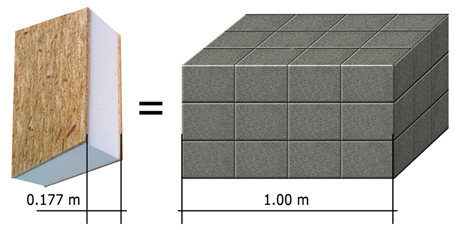# Thermal Resistance

Thermal Resistance (R-value, m2·K/W) is an important parameter that is used in production design and construction. With Thermal resistance we detect appropriate thiknes of outer walls/roof, so that the specific building would meet the regultions of thermal insulation in specific regions and climat zones.Thermal resistance (R-value, m2·K/W) in accordance with EN ISO 10456 and EN ISO 6946 is calculated with the formula:  (d - length(m) of the material; λ-Thermal conductivity ( W/(m · K))

In order to calculate thermal resistance for the base material we use summation method, we sum the individual values of each material’s thermal resistance. For example SIP thickness is 177mm, which consists of a polystyrene plate brand EPS 80, its thickness is 0.153m (λ = 0,0385) and two OSB-3 plates, the thickness of those is 0.012m (λ = 0,13), therefore the R-value for the specific SIP is 4.08m2 · K / W.Comparison:

In order to gain such thermal resistance value for a wall made from concrete blocks, the thickness of walls should be approximately 1 meter (approximate thermal conductivity (λ) of concrete block wall is 0.26).

Tabele of R-values.